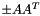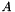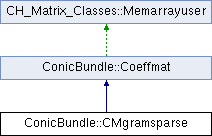ConicBundle
ConicBundle::CMgramsparse Class Reference

implements a Gram matrixas Coeffmat for a sparse rectangular CH_Matrix_Classes::Sparsemat(for use with MatrixSDPfunction, see implemention of a PSCOracle (PSCAffineFunction)). More...

#include <CMgramsparse.hxx>

Inheritance diagram for ConicBundle::CMgramsparse:## Public Member Functions

CMgramsparse (const CH_Matrix_Classes::Sparsemat &Ain, bool pos=true, CoeffmatInfo *cip=0)
copy Ain, the flag for positive/negative and store the user information

virtual Coeffmatclone () const
makes an explicit copy of itself and returns a pointer to it

virtual CH_Matrix_Classes::Integer dim () const
returns the order of the represented symmetric matrix

virtual CH_Matrix_Classes::Real operator() (CH_Matrix_Classes::Integer i, CH_Matrix_Classes::Integer j) const
returns the value of the matrix element (i,j)

virtual void make_symmatrix (CH_Matrix_Classes::Symmatrix &S) const
returns a dense symmetric constraint matrix

virtual CH_Matrix_Classes::Real norm (void) const
returns the Frobenius norm of the matrix

virtual Coeffmatsubspace (const CH_Matrix_Classes::Matrix &P) const
delivers a new object on the heap corresponding to the matrix P^T(*this)P, the caller is responsible for deleting the object

virtual void multiply (CH_Matrix_Classes::Real d)
multiply constraint permanentely by d; this is to allow scaling or sign changes in the constraints

virtual CH_Matrix_Classes::Real ip (const CH_Matrix_Classes::Symmatrix &S) const
returns ip(*this,S)=trace(*this*S), the trace inner product

virtual CH_Matrix_Classes::Real gramip (const CH_Matrix_Classes::Matrix &P) const
returns ip(*this,PP^T)=trace P^T(*this)P

virtual CH_Matrix_Classes::Real gramip (const CH_Matrix_Classes::Matrix &P, CH_Matrix_Classes::Integer start_row, const CH_Matrix_Classes::Matrix *Lam=0) const
returns ip(*this,QQ^T)=trace Q^T(*this)Q for Q=P.rows(start_row,start_row+dim-1)

virtual void addmeto (CH_Matrix_Classes::Symmatrix &S, CH_Matrix_Classes::Real d=1.) const
computes S+=d*(*this);

virtual void addprodto (CH_Matrix_Classes::Matrix &B, const CH_Matrix_Classes::Matrix &C, CH_Matrix_Classes::Real d=1.) const
computes B+=d*(*this)*C

virtual void addprodto (CH_Matrix_Classes::Matrix &B, const CH_Matrix_Classes::Sparsemat &C, CH_Matrix_Classes::Real d=1.) const
computes B+=d*(*this)*C

virtual void left_right_prod (const CH_Matrix_Classes::Matrix &P, const CH_Matrix_Classes::Matrix &Q, CH_Matrix_Classes::Matrix &R) const
computes R=P^T*(*this)*Q

virtual CH_Matrix_Classes::Integer prodvec_flops () const
returns an estimate of number of flops to compute addprodto for a vector

virtual int dense () const
returns 1 if its structure is as bad as its dense symmetric representation, otherwise 0

virtual int sparse () const
returns 0 if not sparse, otherwise 1

virtual int sparse (CH_Matrix_Classes::Indexmatrix &, CH_Matrix_Classes::Indexmatrix &, CH_Matrix_Classes::Matrix &, CH_Matrix_Classes::Real) const
returns 0 if not sparse. If it is sparse it returns 1 and the nonzero structure in I,J and v, where v is multiplied by d. Only the upper triangle (including diagonal) is delivered

virtual int support_in (const CH_Matrix_Classes::Sparsesym &) const
returns 0 if the support of the costraint matrix is not contained in the support of the sparse symmetric matrix S, 1 if it is contained.

virtual CH_Matrix_Classes::Real ip (const CH_Matrix_Classes::Sparsesym &S) const
returns the inner product of the constraint matrix with S

virtual void project (CH_Matrix_Classes::Symmatrix &S, const CH_Matrix_Classes::Matrix &P) const
computes S=P^T*(*this)*P

virtual void add_projection (CH_Matrix_Classes::Symmatrix &S, const CH_Matrix_Classes::Matrix &P, CH_Matrix_Classes::Real alpha=1., CH_Matrix_Classes::Integer start_row=0) const
computes S+=Q^T(*this)Q for Q=P.rows(start_row,start_row+dim-1)

virtual const CH_Matrix_Classes::Matrixpostgenmult (const CH_Matrix_Classes::Matrix &B, CH_Matrix_Classes::Matrix &C, CH_Matrix_Classes::Real alpha=1., CH_Matrix_Classes::Real beta=0., int btrans=0) const
computes C= alpha*(*this)*B^(T if btrans) + beta*C, C is also returned

virtual const CH_Matrix_Classes::Matrixpregenmult (const CH_Matrix_Classes::Matrix &B, CH_Matrix_Classes::Matrix &C, CH_Matrix_Classes::Real alpha=1., CH_Matrix_Classes::Real beta=0., int btrans=0) const
computes C= alpha*B^(T if btrans)*(*this) + beta*C, C is also returned

virtual int equal (const Coeffmat *p, double tol=1e-6) const
returns 1, if p is the same derived class and entries differ by less than tol, otherwise zero

virtual std::ostream & display (std::ostream &o) const
display constraint information

virtual std::ostream & out (std::ostream &o) const
put entire contents onto ostream with the class type in the beginning so that the derived class can be recognized by in().

virtual std::istream & in (std::istream &i)
counterpart to out(), does not read the class type, though. This is assumed to have been read in order to generate the correct class

CMgramsparse (std::istream &is, CoeffmatInfo *cip=0)
constructor with istream and possibly additional user information

const CH_Matrix_Classes::Sparsematget_A () const
returns the const reference to the internal matrix A forming the Gram matrix

bool get_positive () const
returns the flag on whether the Gram matrix is used in positive or negative formPublic Member Functions inherited from ConicBundle::Coeffmat
Coeffmat (bool del_by_CoeffmatPointer=true)
default constructor; set del_by_CoeffmatPointer==true if a CoeffmatPointer reducing the use_cnt to zero should delete this

void set_deletion_by_CoeffmatPointer (bool dbMP)
if set to true any CoeffmatPointer that reduces use_cnt to zero will delete this object

virtual Coeffmattype get_type () const
returns its Coeffmattype

virtual CoeffmatInfoget_info ()
returns the user information

virtual const CoeffmatInfoget_info () const
returns the user information in const form

virtual void set_info (CoeffmatInfo *cip)
deletes the old and sets new user information

## Private Attributes

CH_Matrix_Classes::Sparsemat A
the Coeffmat acts like A*A^T or its negative

bool positive
if true use A*A^T, if false use -A*A^T

## Additional Inherited MembersProtected Member Functions inherited from CH_Matrix_Classes::Memarrayuser
Memarrayuser ()
if memarray is NULL, then a new Memarray is generated. In any case the number of users of the Memarray is incremented

virtual ~Memarrayuser ()
the number of users is decremented and the Memarray memory manager is destructed, if the number is zero.Protected Attributes inherited from ConicBundle::Coeffmat
Coeffmattype CM_type
in order to enable type identification

CoeffmatInfoinfop
allows the user to specify and output additional informationStatic Protected Attributes inherited from CH_Matrix_Classes::Memarrayuser
static Memarraymemarray
pointer to common memory manager for all Memarrayusers, instantiated in memarray.cxx

## Detailed Description

implements a Gram matrixas Coeffmat for a sparse rectangular CH_Matrix_Classes::Sparsemat(for use with MatrixSDPfunction, see implemention of a PSCOracle (PSCAffineFunction)).

While a Gram matrix by itself is always positive semidefinite, there is an extra flag for using its negative version.

The documentation for this class was generated from the following file: